Segmentation via RGB Clustering

Our approach is to explore the computer vision techniques for map separates. This requires more labor and investment than the neural network method which has the advantage of the "black box"-type approach, but one expects that the vision based strategy will be eventually more successful. The disadvantage of the backpropagation approach is that it doesn't leave much space for major improvements-it delivers reasonable quality results for low-level separation but it can hardly be improved by including more insights from higher level reasoning modules since some information is already lost inside the backpropagation "black-box." On the contrary, machine vision is a hierarchical, coupled system with feedback which allows for systematic improvements and provides the proper foundation for the full map understanding program.
The map separates problem translates in the vision jargon into segmentation and region labelling. These algorithms are somewhere on the border of the early and medium vision modules. We have analyzed the RGB clustering algorithm for the map image segmentation. In this method, one first constructs a three-dimensional histogram of the image intensity in the unit RGB cube. For a hypothetical "easy" image composed of a small, fixed number of colors, only a fixed number of histogram cells will be populated. By associating distinct labels with the individual nonempty cells, one can then filter the image, treating the histogram as a pixellabel look-up table-that is, assigning for each image pixel the corresponding cell label. For "real world" map images involving color fluctuation and diffusion across region boundaries, the notion of the isolated histogram cells should be replaced by that of the color clusters. The color image segmentation algorithm first isolates and labels individual clusters. The whole RGB cube is then separated into a set of nonoverlapping polyhedral regions, specified by the cluster centers. More explicitly, for each histogram cell, a label is assigned given by the label of the nearest cluster, detected by the clustering algorithm. The pixelregion look-up table constructed this way is then used to assign region labels for individual pixels in the image.
As a first test of the RGB clustering, we constructed a set of color histograms with fixed bin sizes and various resolutions (Figure 17.12). Even with such a crude tool, one can observe that the clustering method is very efficient for most image regions and when appropriately extended to allow for irregular bin volumes, it will provide a viable segmentation and labelling algorithm. The interactive tool in Figure 17.12 also provided a nice test of rapid prototyping capabilities of MOVIE. The whole MovieScript code for the demo is on the order of only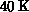and it was created interactively based on the integrated scriptable tools for Motif, field algebra, and imaging.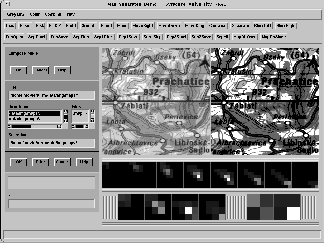Figure 17.12: Map Separates Tool Constructed in MovieScript in the Rapid Prototyping Mode to Test the RGB Clustering Techniques. The left image represents the full color source, the right image is separated into a fixed number of base colors. Three RGB histograms are constructed with the bin sizes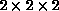,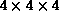, and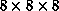, correspondingly. Each histogram is represented as a sequence of RG planes, parametrized by the B values. The first row under the image panel contains eight blue planes of thehistogram, the second row containsandhistograms. The content of each bin is encoded as an appropriate shade of gray. A mouse click into the selected square causes the corresponding separate to be displayed in the right image window, using the average color in the selected bin. In the separate mode, useful for previewing the histogram content, subsequent separates overwrite the content of the right window. In the compose mode, used to generate this snapshot, subsequent separates are superimposed. Tools are also provided for displaying all separates for a given histogram in the form of an array of images.

The simple, regular algorithm in Figure 17.12 cannot cope with problems such as shaded relief, non-convex clusters, and color ambiguities, discussed above. To handle such problems, we need interactive tools to display, manipulate, and process three-dimensional histograms. The results presented below were obtained by coupling MOVIE field algebra with the AVS visualization modules. In preparation is the uniform MovieScript-based tool with similar functionality, exploiting the currently developed support for the three-dimensional graphics in MOVIE.
The RGB histogram for the ad250 image is presented in Figure 17.13. Each nonempty histogram cell is represented as a sphere, centered at the bin center, with the radius proportional to the integrated bin content and with the color given by the average RGB value of this cell. Poor color separation manifests as cluster concentration along the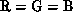axis. Two large clusters along this diagonal correspond to white and grey patches on the image. A "pipe" connecting these two clusters is the effect of shaded relief, composed of a continuous band of shades of gray. Three prominent off-diagonal clusters, forming a strip parallel to the major white-gray structure, represent two tints of true green and dark green, again with the shaded relief "pipe." Brown isoclines are represented by an elongated cloud of small spheres, scattered along the white-gray structure.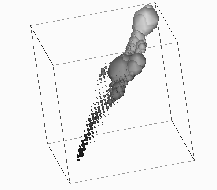Figure: Color histogram of the ad250 image (see Figure 17.10) in the unit RGB cube. Histogram resolution is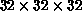. Each bin is represented by a sphere with the radius proportional to the bin content and with the average color value in this bin.

The separation of these three elongated structures-white, green, and brown-represents the major complexity since all three shapes are parallel and close to each other. The histogram in Figure 17.13 is constructed with theresolution, which is slightly too low for numerical analysis (discussed below) but useful for graphical representation as a black-and-white picture. The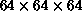histogram, used in actual calculations, contains too many small spheres to create any compelling three-dimensional sensation without the color cues and interactive three-dimensional tools (however, it looks impressive and spectacular on a full-color workstation with 3D graphics accelerator). By working interactively with thehistogram, one can observe that all three major clusters are in fact reasonably well separated.
All remaining colors separate in an easy way: Shades of black again form a scattered diagonal strip which is far away from the three major clusters and separates easily from a similar, smaller parallel strip of shades of purple; red separates as a small but prominent cluster (close to the central gray blob in Figure 17.13); finally, blue is very dispersed and manifests as a broad cloud or dilute gas of very small spheres, invisible in Figure 17.13 but again separating easily into an independent polyhedral sector of the RGB cube.
The conclusion from this visual analysis, only partially reproduced by the picture in Figure 17.13, is that RGB clustering is the viable method for separating ad250 into the seven indicated base colors. As mentioned above, this separation process requires human guidance because of the color mapping ambiguities. The nontrivial technical problem from the domain of human-machine interface we are now facing is how to operate interactively on complex geometrical structures in the RGB cube. A map analyst should select individual clusters and assign a unique label/color with each of them. As discussed above, these clusters are separable but their shapes are complex, of them given as clouds of small spheres, some others elongated, non-convex, and so on.
Virtual reality-type interface with the glove input and the three-dimensional video output could offer a natural solution for this problem. For example, an analyst could encircle a selected cluster by a set of hand movements. Also, the analyst's presence inside the RGB cube, implemented in terms of the immersive video output, would allow for fast and efficient identification of various geometric structures.
Right now, we adopted a more cumbersome but also more realistic approach, implementable in terms of conventional GUI tools. Rather than separate clusters, we reconstruct them from a set of small spheres. An interactive tool was constructed in which an analyst can select a small region or even a single pixel in the image and assign an effective color/label to it. This procedure is iterated some number of times. For example, we click into some white areas and say: white. Then we click into few levels of a shaded relief and we say again: white. Finally, we click into the gray region and we also say: white. In a similar way, we click into some number of isoclines with various tints of brown and we say: brown. Each point selected in this way becomes a center of a new cluster.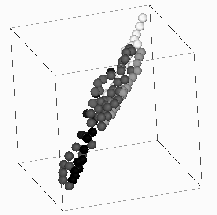Figure 17.14: A set of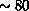Color Values, Selected Interactively and Mapped on the Specified Set of Seven Base Colors as Described in the Text

The set of clusters selected this way defines the partition of the RGB cube into a set of nonoverlapping polyhedral regions. Each such region is convex and therefore the number of small clusters to be selected in this procedure must be much larger than the number of "real" clusters (which is seven in our case), since the real clusters often have complex, nonconvex shapes.
A sample selection of this type is presented in Figure 17.14. It contains about 80 small spheres, each in one of the seven base colors. Separation of "easy" colors such as blue or red can be accomplished in terms of a few clusters. Complex structures such as white, green, and brown require about 20 clusters each to achieve satisfactory results. The image is then segmented using the color look-up table constructed in this way and the weight is assigned to each small cluster, proportional to the integrated content of the corresponding polyhedral region. The same selection as in Figure 17.14, but now with the sphere radii proportional to this integrated content, is presented in Figure 17.15.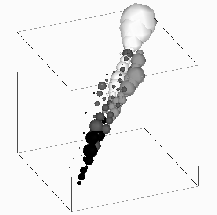Figure: The Same Set of Selected Color Values as in Figure 17.14 But Now with the Radius Proportional to the Integrated Content of Each Polyhedral Cell with the Center Specified by the Selected RGB Value.

As seen, we have effectively reconstructed a shape with topology roughly similar to the original histogram in Figure 17.13 but now separated into the seven base colors.
The resulting separated image is presented in Figure 17.16 and compared with the JPL result in Figure 17.11 in the next section.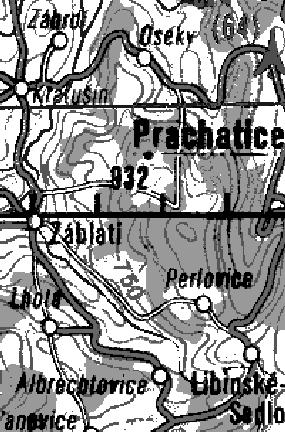Figure: Image ad250 from Figure 17.10, Separated into Seven Base Colors Using the RGB Clustering Algorithm with the Clusters and Colors Selected as in Figure 17.15

Project Report

Computational Geometry CPSC 516
vk@cs.ubc.ca

Pixel Clustering Methods in RGB space

UBC
December 1998

Content

1. Introduction to cluster analysis
2. Literature review
3. Project scope
4. Description of the clustering algorithms

4.1 Bits Per Colour resolution algorithm (BPC)
4.2 Octree algorithm
4.3 Greedy algorithm
4.4 K-means algorithm
4.5 Continuous K-means algorithm (R-means)

5. Algorithms performance comparison
6. Conclusion
7. Hardware and Software
8. References

1. Introduction to cluster analysis

Clustering problems arise in many disciplines and have a wide range of applications, such as data compression, information retrieval, computer vision and data mining. Clustering techniques seek to separate a set of data into groups or clusters and may be classified into types roughly as follows :

a. Hierarchical techniques - in which the clusters themselves are classified into groups, the process being repeated at different levels to form a tree.

b. Optimization-partitioning techniques - in which the clusters are formed by optimization of a 'clustering criterion'. The clusters are mutually exclusive, thus forming a partition of the set of entities.

c. Density or mode-seeking techniques - in which clusters are formed by searching for regions containing a relatively dense concentration of entities.

d. Clumping techniques - in which the clusters or clumps can overlap.

e. Others

Many attempts have been made to propose a formal definition of a term cluster but non of them has claimed to provide a universal definition. One states that cluster is a group of contiguous elements of a statistical population. Other definitions contain statements such as 'a cluster is a set of entities which are alike, and entities from different clusters are not alike'. Other suggest that entities within a cluster are more similar to each other then the entities from another cluster.

A description of what constitutes a cluster which probably agrees closely with our intuitive understanding of the term is given by considering entities as points in a p-dimensional space, with each of the p variables being represented by one of the axis of this space. The variable values for each entity define a p dimensional coordinate in this space. Clusters may now be described as continuous regions of this space containing a relatively high density of points, separated from other such regions by regions containing a relatively low density of points. This description matches the way we detect clusters visually in two or three dimensional space.

One of the most familiar applications of clustering is the classification of plants or animals into distinct groups or species. However, the main purpose of clustering data is to reduce the size and complexity of the data set. Data reduction is accomplished by replacing the coordinates of each point in a cluster with the coordinates of that cluster's reference point. Clustered data require considerably less storage space and can be manipulated more quickly than the original data. The value of a particular clustering method depends on how closely the reference points represent the data as well as how fast the program runs.

What determines a "good," or representative, clustering? Consider a single cluster of points along with its centroid or mean. If the data points are tightly clustered around the centroid, the centroid will be representative of all the points in that cluster. The standard measure of the spread of a group of points about its mean is the variance, often the sum of the squares of the distance between each point and the mean. If the data points are close to the mean, the variance will be small. A generalization of the variance, in which the centroid is replaced by a reference point that may or may not be a centroid, is used in cluster analysis to indicate the overall quality of a partitioning.

All types of clustering techniques have various problems.

Hierarchical techniques have a general disadvantage since they contain no provision for reallocation of entities who may have been poorly classified at an early stage in the analysis.

Optimization techniques, which seek to optimize some criterion have a problem of finding a sub-optimal solution instead of a global solution. This is known as a local optima problem. Most of them also presume that the number of clusters is known or given prior to clustering.

Density seeking methods, such as fitting mixtures of multivariate normal distributions also suffer from the problem of sub-optimal solutions, since there may be more than one solution to the maximum likelihood equations.

Other techniques have their own problems, e.g. presuming that points form a hyper-spherical clusters, what may not be the case in many situations.

Therefore, the investigator who decides to use cluster analysis techniques, and has a large number of methods available to him, must examine the assumptions made by a particular method and satisfy himself that such assumptions are reasonable for his particular data. Because different techniques are likely to give different solutions, he should consider the validity of any found clusters and try various methods with the data to select the one giving best results.

2. Literature review

The fundamentals of cluster analysis, as well as a good review of the classical clustering techniques, are given by B. Everitt in . L. Kaufman and P. Rousseeuw provide in  a brief review but detailed programming examples of six common clustering methods. An interesting, simple and efficient, clustering algorithm called a 'greedy algorithm' was suggested by T. Gonzales in  and later improved by T. Feder D. Greene in . Further development of this method may be found in the work by P. Agraval and C. Procopiuc . Another simple and efficient algorithm for clustering data, very popular 'k-means' algorithm, was conceptually described by S.P. Lloyd in  and later improved by J. MacQueen in . Many papers as [2,4,10,14] suggest various improvements to the k-means algorithm, mostly based on the usage of the random sub-sampling of the data sets at various stages of the algorithm. Since both 'greedy' and 'k-means' assume that k, the number of clusters, is given prior to clustering, and clusters have a hyper-spherical shape, for practical implementation I also suggest the extended version of a 2D quad-tree clustering method first suggested by K. Fu and R. Gonzalez in  and described by R. Gonzalez and R. Woods in . This method is applied in a sense of quantizing image colour and merging the adjacent clusters.

3. Project scope

Many types of data analysis, such as the interpretation of colour images involve data sets sometimes excessively large that their direct manipulation is impractical. Some method of data compression or consolidation and region segmentation must first be applied to reduce the size of the data set without losing the essential character of the data and the context of the image.

All consolidation methods sacrifice some detail and many segmentation methods misinterpret some regions. The most desirable methods have to be computationally efficient and yield results that are at least for practical applications representative of the original data and image context.

In this project we implement several widely used algorithms that consolidate data by clustering and segment image by regions, Greedy, K-means, Continuous K-means, and then present two new methods, developed by the author, BPC and Octree. We also provide a comparative analysis of the algorithms performance.

4. Description of the clustering algorithms

4.1 Bits Per Colour resolution algorithm (BPC), due to the author

Given S a set of N discrete points in 3D (RGB pixels of the image, 8 bits per colour, 24 bits per pixel), the procedure divides pixels into clusters by reducing, for the divisive version, or increasing, for the agglomerative version, each colour resolution by one at a step.

The geometrical interpretation of the algorithm is as follows:

Agglomerative version

- 0 bits per colour (bpc), all pixels are in one cluster and are of the same colour which is represented by a single point in the center of the RGB cube

- 1 bpc, now we have 2*2*2 = 8 possible clusters (colours), what can be interpreted as splitting the RGB cube into 8 sub-cubes (each dimension is divided into two equal parts). The original RGB pixels are scaled into the obtained model. The number of output clusters is dependent on the initial distribution of pixels. The colour of a cluster is determined by the center of the sub-cube that represents a particular cluster. The colour of a pixel is determined by a sub-cube into which it has been scaled.

- 2 bpc, now we have 4*4*4 = 64 possible clusters (colours), what can be interpreted as splitting the RGB cube into 64 sub-cubes (each dimension is divided into four equal parts). The original RGB pixels are scaled into the obtained model. The number of output clusters is dependent on the initial distribution of pixels. The colour of a cluster is determined by the center of the sub-cube that represents a particular cluster. The colour of a pixel is determined by a sub-cube into which it has been scaled. the rest of the steps are analogous and we will provide only the numbers

- 3 bpc, 512 possible clusters
- 4 bpc, 4,096 possible clusters
- 5 bpc, 32,768 possible clusters
- 6 bpc, 262,144 possible clusters
- 7 bpc, 2,097,152 possible clusters
- 8 bpc, 16,777,216 possible clusters

At full colour resolution we have 8 bits per colour or 256 possible variations of a single colour. For three colours (RGB) this is equivalent to 16,777,216 possible pixel values.

A few words about scaling a pixel into the center of a corresponding sub-cube. Given REZ - a colour resolution at the current step and a pixel value in a form of 3 bytes (RGB) the scaling can be done in a constant time as follows:

zero REZ lower bits of byte R and add a byte (128 >> REZ)
zero REZ lower bits of byte G and add a byte (128 >> REZ)
zero REZ lower bits of byte B and add a byte (128 >> REZ)

where >> designates a right shift operation

Therefore, the BPC algorithm runs in O(N) time consuming O(1) memory.

The divisive version of the algorithm is similar to the agglomerative one, but steps are taken in opposite order.

4.2 Octree algorithm, due to the author

The algorithm may be applied to the results of the BPC algorithm.

Given S a set of N discrete points in 3D (RGB pixels of the image, 8 bits per colour, 24 bits per pixel), the procedure merges the adjacent pixels (sub-cubes of the RGB cube) into a single cluster. Pixels in the cluster get a colour of the cluster centroid.

Given enough computer memory, in case of the full colour (8 bpc) we need

 a. a global Look Up Table for all possible pixel values 3 * 2^^(REZ*3) bytes b. to process centroids of each cluster we need 5 * N bytes c. to mark pixels being clustered we need two aux. LUTs 2^^(REZ*3) bits each

the algorithm may be designed to run in O(N) time consuming O(2^^REZ) or O(N) memory, whatever is bigger.

Procedure works as follows:

a. It maps N pixels of the image into the first aux. LUT, by setting the corresponding bits of the LUT to 1s. Each possible pixel value is represented by 1 bit in the LUT. A 2 Mb LUT can describe a space of all possible values of RGB pixels at 8 bpc.

b. Copies the first aux. LUT into the second aux. LUT.

c. For each pixel in the image, procedure checks if the pixel has already been clustered, by checking a particular bit of the second aux. LUT. If yes, the current cluster number is taken from the global LUT. If not, the pixel is assigned a new cluster number which is placed into a particular section of the global LUT and the pixel is marked as clustered by setting a particular bit of the second aux. LUT to 0.

d. If adjacent pixels (sub-cubes of the RGB cube) of the current pixel are present in the image, we can check this by checking the value of the corresponding bit in the first aux. LUT, we mark those adjacent pixels as belonging to the current pixel cluster, by placing the current cluster number into the corresponding global LUT sections. If any of those adjacent pixels have already been clustered, we cluster them anyway as belonging to the current cluster and divert their old clusters to the new cluster (we may use a table for processing centroids temporarily for this purpose). By diverting old clusters to new clusters we merge clusters.

e. Calculate centroids for each cluster and colour the image pixels with cluster colours.

4.3 Greedy algorithm, due to T. Gonzalez 

Given S a set of N points and K the number of clusters, the procedure chooses, in a greedy manner, a subset H of S consisting of K points that are farthest apart from each other and maintains for every point p of (S | H) the value dist(p) = min d(p,q), where q is a point of H and d(p,q) is the distance between p and q in the underlying metric (in our implementation we used the Euclidian distance between points). Each point h of H determines a cluster, denoted cluster(i). A point p of (S | H) is assigned to cluster(i) if it is closer to h(i) than to any other point in H.

 H = {p(1)} /* p(1) is a random point of S */ cluster(0) = S for i = 1 to N dist(s(i)) = d(p(i), p(1)) for i = 1 to K-1 { D = max {dist(p) where p is a point of (S | H)} choose h(i) of (S | H), where dist(h(i)) = D H = H U {h(i)} for j = 1 to N { if d(s(j), h(i)) <= dist(s(j)) { dist(s(j)) = d(s(j), h(i)) cluster(i) = cluster(i) U {s(j)} } } }

The running time of the algorithm is easily determined to be O(N*K). It has been later improved to O(N*logK) by Feder and Greene .

4.4 K-means algorithm, due to S. Lloyd  and J. MacQueen 

Given S a set of N points and K the number of clusters, the procedure

a. arbitrarily chooses K reference points from S (in our implementation we choose the reference points at random). Each reference point defines a cluster.

b. then data points are partitioned into K clusters. Point p of S becomes a member of cluster(i) if p is closer to the reference point of cluster(i) than to any other reference point (in our implementation we used Euclidean distance to determine the proximity).

c. the centroid for each cluster is calculated and the centroid becomes a reference point of its cluster.

d. then, during successive iterations, the centroids of each cluster are adjusted.

During the iteration we go through all the data points and determine if for point p in cluster(i), the centroid of cluster(i) is the nearest reference point. If so, no adjustments are made and the algorithm proceeds to the next data point. However, if the centroid of cluster(j) becomes the reference point closest to the data point p, then p is reassigned to cluster(j), the centroids of the loosing cluster(i) (minus point p) and the gaining cluster(j) (plus point p) are recomputed, and the reference points of clusters i and j are moved to their new centroids. After each iteration, every one of the K reference points is a centroid, or mean, hence the name "k-means".

The iterations proceed until, for all data points, no re-allocation of points from cluster to cluster is registered.

Finally, the distribution of points will correspond to the centroidal Voronoi configuration, where each data point is closer to the reference point of its cluster than to any other reference point, and each reference point is the centroid of its cluster.

The algorithm is similar to the fitting routine, which begins with an initial guess for each fitted parameter and then optimizes their values. The algorithm runs in O(N*K) time.

The K-means algorithm does not guarantee the best possible partitioning, or finding the global minimum in terms of the error measure, but only provides a local minimum. However, the improvement of the partitioning and the convergence of the error measure to a local minimum is often quite fast, even when the initial reference points are badly chosen.

4.5 Continuous K-means algorithm (R-means), due to Vance Faber 

The R-means is similar to the standard K-means algorithm described above but differs from it in how the initial reference points are chosen and how data points are selected for the centroid updating process.

In the standard K-means, the initial reference points are chosen more or less arbitrarily. In the R-means reference points are chosen as a random sample from the whole population of data points. If sample is sufficiently large, the distribution of these initial reference points should reflect the distribution of points in the entire set. If the whole set of points is densest in a particular region, then the sample should also be densest in that region.

Another difference in the standard and continuous k-means algorithms is the way the data points are treated. During each complete iteration, the standard algorithm examines all the data points in sequence. In contrast, the continuous algorithm examines only a random sample of data points. If the data set is large and the sample is representative of the data set, the algorithm converges much more quickly than an algorithm that examines every point in sequence.

These modifications to the standard algorithm greatly accelerate the clustering process. Since both the reference points and the data points for the updates are chosen by random sampling, more reference points will be found in the densest regions of the data set and the reference points will be updated by data points in the most critical regions. When applied to a large data set, the algorithm normally converges to a solution after only a small fraction of the total points have been examined. This rapid convergence distinguishes the continuous k-means from less efficient algorithms but the algorithm still has the same time complexity of O(N*K).

5. Algorithms performance comparison

The results of the algorithms performance tests on clustering pixels of live sequence of images may be found in the below table. Results are split into nine categories and reflect both qualitative and quantitative analysis.

 BPC Octree Greedy K-means R-means Running time excellent excellent good average good Retaining image context excellent good good excellent good Data compression excellent average excellent excellent excellent Visual colour precision good average good good good Distant colour separation average excellent excellent average average Prime image colour description good average good excellent excellent Small region description good average good excellent excellent Object colour stability excellent poor good poor poor

6. Conclusion

In this project we have implemented 5 clustering algorithms and tested their performance on clustering pixels of the live image sequence. The project, that has a significant computational geometry component, also serves a double duty. Its results may be applied to such areas as computer vision and pattern recognition. Many problems in computer vision, for instance, can be formulated as clustering problems, and among the most common is the segmentation of multidimensional images.

One of the interesting aspects learned in the project is how the running time of the algorithm (time complexity) depends on the amount of memory used (space complexity). During the project, the same algorithm have been implemented in different ways, with different time and space complexities. For instance, the algorithm of counting how many different clusters are there in the image went trough evolution of O(N^^2), then output sensitive O(N*K), and finally O(N) running time, where N is the number of pixels and K the number of clusters, but every time it consumed more and more memory.

Another interesting aspect learned is how the incorporation of random data sub-sampling can speed up the algorithm. Some time the speed up is gained at a cost of clustering quality, but by large the usage of random techniques is beneficial.

All implemented clustering algorithms worked well but non of them could be classified as an ultimate leader. Depending on the purpose of clustering, and the definition of the cluster itself, a particular method should be selected. There were more expectations about the Octree method, especially that it can merge pixels of the smooth colour/intensity gradient into one single cluster. It does not seem to work that way on real images, most likely because of the cluster spacial discontinuities in the RGB space. To overcome this problem, less fine colour resolution have been applied, but it caused other clusters, that by image context should not merged with the considered cluster, to merge with it. The cluster colour constancy is also a bit of a trouble because clusters merge and separate form one image to another and the centroids move around quite irregular. One good feature of the Octree, where it outperformed other algorithms, is the separation of the geometrically distant clusters. In the image sense it can be interpreted as segmenting out bright spots that don't merge with the overall background colour. The BPC method turned out to be very efficient in general. Other methods worked quite well in their own categories.

The project is implemented in a way that the results of clustering can be visually observed and evaluated by a viewer.

7. Hardware and Software

The clustering algorithms have been implemented and real time capabilities checked using the following

equipment

Pentium II 233 Mhz, 150 Meg RAM, PC
Matrox Meteor frame grabber
Sony EVI D30 colour camera

and software

Linux OS
GCC compiler
Motif libraries

The program consists of the following blocks (~1,500 lines of code)

shift.cc assembly language routines for

- calculating the number of possible pixel values at the current resolution
- reducing the colour resolution of the entire image using MMX technology
- checking the bit setting in the RGB model LUT
- setting the bit in the RGB model LUT
- clearing the bit in the RGB model LUT
- zeroing a block of memory
- copying a block of memory

measure.cc C and assembly routines for

- reading the CPU clock counter
- calculating the difference between the counter readings
- converting CPU ticks into milliseconds

seg.cc main program

- grabs frames from the camera
- clusters pixels of the image using various clustering algorithms
- BPC
- Octree
- Greedy
- Kmeans
- Rmeans
- calculates the number of different clusters in the image
- displays the results of clustering on the screen

8. References

 P.K. Agarwal, C.M. Procopiuc, Exact and Approximation Algorithms for Clustering, Proceedings of the 9th ACM-SIAM Symposium on Discrete Algorithms, 1998.

 P.S. Bradley, Usama M. Fayyad, Refining Initial Points for K-Means Clustering, To Appear in: Proceedings of the Fifteenth International Conference on Machine Learning, Morgan Kaufmann, July 1998

[N/A] R.O. Dabes, A.K. Jain, Algorithms for Clustering Data, Prentice Hall, 1988

 B. Everitt, Cluster Analysis, Heinemann Educational Books, 1974

 V. Faber, Clustering and the Continuous k-Means Algorithm, Los Alamos Science Magazine, Number 22, 1994.

 T. Feder and D. Greene, Optimal algorithms for approximate clustering. Proc. 20th Annual ACM Sympos. Theory Comput., 1988

 E. Forgy, Cluster analysis of multivariate data: efficiency vs. interpretability of classifications, Biometrics 1965

 K. Fu, R. Gonzalez, C. Lee, Robotics, Control, Sensing, Vision and Intelligence, McGraw-Hill, NY, 1987

 R. Gonzalez, R. Woods, Digital Image Processing, Addison Wesley, 1992

 T. Gonzalez, Clustering to minimize the maximum inter-cluster distance, Theoretical Computer Science #38, 1985 [N/A] J. Hartigan, Clustering Algorithms, John Wiley & Sons, 1975

 M. Inaba, H. Imai, M. Nakade, T. Sekiguchi, Application of an Effective Geometric Clustering Method to the Color Quantization problem, 13th ACM symposium on Computational Geometry, June 1997

 L. Kaufman, P.J. Rousseeuw, Finding Groups in Data: An Introduction to Cluster Analysis, John Wiley & Sons, 1990

 S.P. Lloyd. 1982. Least squares quantization in PCM. IEEE Transactions on Information Theory IT-28: 129 137.

 J. MacQueen, Some methods for classification and analysis of multivariate observations, Proceedings of the Fifth Berkeley Symposium on Mathematical Statistics and Probability. Volume I, Statistics. University of California Press, 1967

 S. Schroter, Lookup-Table Calibration for Efficient Colour Image Segmentation, Forschungsbericht 666/1998, Fachbereich Informatik, Universitat Dortmund, 1998